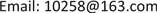1. 引言

2. 分析模型

V 1 = 4 3 π r 3 (2)

E B 1 = E S 1 + E L 1 = γ S A 1 + γ L S 1 (3)

S 2 = 2 π R 2 ( 1 − cos θ ) (4)

V 2 = 1 3 π R 3 ( 1 − cos θ ) 2 ( 2 + cos θ ) (5)

A 2 = π ω 2 = π R 2 sin 2 θ (6)

E B 2 = E S 2 + E L 2 + E L S 2 = γ S ( A 1 − A 2 ) + γ L S 2 + γ L S A 2 (7)

W N = ( γ L + γ S − γ S L ) A 2 (8)

4 r 3 R 3 = ( 1 − cos θ ) 2 ( 2 + cos θ ) (9)

E B 3 = E S 3 + E L 3 = γ S A 1 + γ L ( S 2 + A 2 ) (10)

E X 3 − E X 1 = E B 1 − E B 3 = γ L ( S 1 − S 2 − A 2 ) (11)

3. 表面能

E B 1 + E X 1 = E B 2 + E X 2 + E N (12)

W B 1 = ∫ 0 A 2 ( γ S − γ S L − γ L cos θ ) d A 2 (13)

γ L cos θ P = γ S − γ S L (14)

W B 1 = [ 1 − 2 − cos θ P ( 1 + cos θ ) 4 ( 1 − cos θ ) ( 2 + cos θ ) 2 3 ] E L 1 = − ( E B 2 − E B 1 ) (15)

W B 1 = ( 1 − r R p ) E L 1 (16)

F = γ S − γ S L − γ L cos θ (16a)

4. 压力能

E Y 1 = ∫ d ( P 1 V 1 ) = 2 γ L r × 4 3 π r 3 = 2 3 E L 1 (17)

E Y 2 = ∫ d ( P 2 V 1 ) = 2 γ L R × 4 3 π r 3 = 2 r 3 R E L 1 (18)

W B 2 = − ( E Y 2 − E Y 1 ) = 2 3 ( 1 − r R ) E L 1 (19)

W B = W B 1 + W B 2 = 5 3 ( 1 − r R P ) E L 1 (20)

5. 粘性损耗

W ϕ = W B 2 = 2 3 ( 1 − r R ) E L 1 (21)

W ϕ = Δ E 动 能 + Δ E 表 面 能 (22)

6. 结论

1) 液滴铺展过程中，内能守恒，表面能、粘附能和变形能相互转化，没有参与粘性耗散；

2) 粘性耗散的主要能量来源于压力能；

3) 表面张力的水平分量做功引起了内能的转化，垂直分量做功引起了粘性耗散。